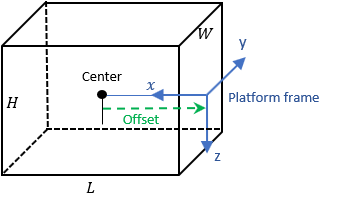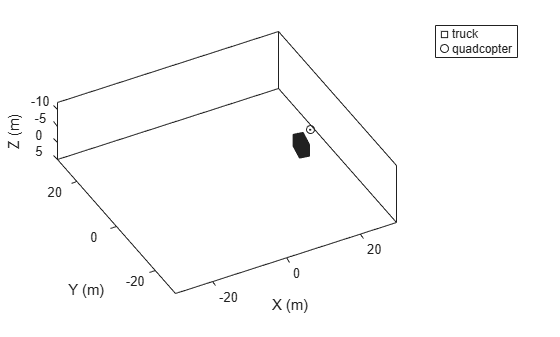# Platform

Platform object belonging to tracking scenario

Since R2018b

## Description

`Platform` defines a platform object belonging to a tracking scenario. Platforms represent the moving objects in a scenario and are modeled as points or cuboids with aspect-dependent properties.

## Creation

You can create `Platform` objects using the `platform` method of `trackingScenario`.

## Properties

expand all

Scenario-defined platform identifier, specified as a positive integer. The scenario automatically assigns `PlatformID` values to each platform.

Data Types: `double`

Platform classification identifier specified as a nonnegative integer. You can define your own platform classification scheme and assign `ClassID` values to platforms according to the scheme. The value of `0` is reserved for an object of unknown or unassigned class.

Example: `5`

Data Types: `double` | `single`

Current position of the platform, specified as a 3-element vector of scalars.

• When the `IsEarthCentered` property of the scenario is set to `false`, the position is expressed as a three element Cartesian state [`x`, `y`, `z`] in meters.

• When the `IsEarthCentered` property of the scenario is set to `true`, the position is expressed as a three element geodetic state: `latitude` in degrees, `longitude` in degrees, and `altitude` in meters.

The position is determined by the platform trajectory defined in the `Trajectory` property.

Data Types: `double`

Current orientation of the platform, specified as a 3-element vector of scalars in degrees. The three scalars are the [`yaw`, `pitch`, `roll`] rotation angles from the local reference frame to the platform's body frame. The orientation is determined by the platform trajectory defined in the `Trajectory` property.

Data Types: `double`

Platform dimensions and origin offset, specified as a structure. The structure contains the `Length`, `Width`, `Height`, and `OriginOffset` of a cuboid that approximates the dimensions of the platform. The `OriginOffset` is the position vector from the center of the cuboid to the origin of the platform coordinate frame. The `OriginOffset` is expressed in the platform coordinate system. For example, if the platform origin is at the center of the cuboid rear face as shown in the following figure, then set `OriginOffset` as `[-L/2, 0, 0]`. The default value for `Dimensions` is a structure with all fields set to zero, which corresponds to a point model.Fields of `Dimensions`

FieldsDescriptionDefault
`Length`Dimension of a cuboid along the x direction`0`
`Width`Dimension of a cuboid along the y direction`0`
`Height`Dimension of a cuboid along the z direction`0`
`OriginOffset`Position of the platform coordinate frame origin with respect to the cuboid center`[0 0 0 ]`

Example: `struct('Length',5,'Width',2.5,'Height',3.5,'OriginOffset',[-2.5 0 0])`

Data Types: `struct`

Platform motion, specified as either a `kinematicTrajectory` object, a `waypointTrajectory` object, or a `geoTrajectory` object. The trajectory object defines the time evolution of the position and velocity of the platform frame origin, as well as the orientation of the platform frame relative to the scenario frame.

Platform signatures, specified as a cell array of `irSignature`, `rcsSignature`, and `tsSignature` objects or an empty cell array. The cell array contains at most only one instance for each type of signature objects listed. A signature represents the reflection or emission pattern of a platform such as its radar cross-section, target strength, or IR intensity.

A pose estimator, specified as a pose-estimator object such as `insSensor`. The pose estimator determines platform pose with respect to the local NED scenario coordinate. The interface of any pose estimator must match the interface of `insSensor`. By default, pose-estimator accuracy properties are set to zero.

Emitters mounted on platform, specified as a cell array of emitter objects, such as `radarEmitter` or `sonarEmitter`.

Sensors mounted on platform, specified as a cell array of sensor objects such as `irSensor`, `fusionRadarSensor`, `monostaticLidarSensor`, or `sonarSensor`.

Mesh of platform, specified as an `extendedObjectMesh` object. The object represents the mesh as vertices and faces. The `monostaticLidarSensor` object uses the platform mesh information to generate cloud data.

## Object Functions

 `detect` Detect signals using platform-mounted sensors `lidarDetect` Report point cloud detections from all lidar sensor on platform `emit` Radiate signals from emitters mounted on platform `pose` Pose of platform `targetPoses` Target positions and orientations as seen from platform `targetMeshes` Target meshes as seen from platform

## Examples

collapse all

Create a tracking scenario and a platform following a circular path.

```scene = trackingScenario('UpdateRate',1/50); % Create a platform plat = platform(scene); % Follow a circular trajectory 1 km in radius completing in 400 hundred seconds. plat.Trajectory = waypointTrajectory('Waypoints', [0 1000 0; 1000 0 0; 0 -1000 0; -1000 0 0; 0 1000 0], ... 'TimeOfArrival', [0; 100; 200; 300; 400]); % Perform the simulation while scene.advance p = pose(plat); fprintf('Time = %f ', scene.SimulationTime); fprintf('Position = ['); fprintf('%f ', p.Position); fprintf('] Velocity = ['); fprintf('%f ', p.Velocity); fprintf(']\n'); end```
```Time = 0.000000 ```
```Position = [ ```
```0.000000 1000.000000 0.000000 ```
```] Velocity = [ ```
```15.707701 -0.000493 0.000000 ```
```] ```
```Time = 50.000000 ```
```Position = [ ```
```707.095476 707.100019 0.000000 ```
```] Velocity = [ ```
```11.107152 -11.107075 0.000000 ```
```] ```
```Time = 100.000000 ```
```Position = [ ```
```1000.000000 0.000000 0.000000 ```
```] Velocity = [ ```
```0.000476 -15.707961 0.000000 ```
```] ```
```Time = 150.000000 ```
```Position = [ ```
```707.115558 -707.115461 0.000000 ```
```] Velocity = [ ```
```-11.107346 -11.107341 0.000000 ```
```] ```
```Time = 200.000000 ```
```Position = [ ```
```0.000000 -1000.000000 0.000000 ```
```] Velocity = [ ```
```-15.707963 0.000460 0.000000 ```
```] ```
```Time = 250.000000 ```
```Position = [ ```
```-707.098004 -707.098102 0.000000 ```
```] Velocity = [ ```
```-11.107069 11.107074 0.000000 ```
```] ```
```Time = 300.000000 ```
```Position = [ ```
```-1000.000000 0.000000 0.000000 ```
```] Velocity = [ ```
```-0.000476 15.707966 0.000000 ```
```] ```
```Time = 350.000000 ```
```Position = [ ```
```-707.118086 707.113543 0.000000 ```
```] Velocity = [ ```
```11.107262 11.107340 0.000000 ```
```] ```
```Time = 400.000000 ```
```Position = [ ```
```-0.000000 1000.000000 0.000000 ```
```] Velocity = [ ```
```15.708226 -0.000493 0.000000 ```
```] ```

Create a tracking scenario with two cuboid platforms following circular trajectories.

```sc = trackingScenario; % Create the platform for a truck with dimension 5 x 2.5 x 3.5 (m). p1 = platform(sc); p1.Dimensions = struct('Length',5,'Width',2.5,'Height',3.5,'OriginOffset',[0 0 0]); % Specify the truck's trajectory as a circle with radius 20 meters. p1.Trajectory = waypointTrajectory('Waypoints', [20*cos(2*pi*(0:10)'/10)... 20*sin(2*pi*(0:10)'/10) -1.75*ones(11,1)], ... 'TimeOfArrival', linspace(0,50,11)'); % Create the platform for a small quadcopter with dimension .3 x .3 x .1 (m). p2 = platform(sc); p2.Dimensions = struct('Length',.3,'Width',.3,'Height',.1,'OriginOffset',[0 0 0]); % The quadcopter follows the truck at 10 meteres above with small angular delay. % Note that the negative z coordinates correspond to positive elevation. p2.Trajectory = waypointTrajectory('Waypoints', [20*cos(2*pi*((0:10)'-.6)/10)... 20*sin(2*pi*((0:10)'-.6)/10) -11.80*ones(11,1)], ... 'TimeOfArrival', linspace(0,50,11)');```

Visualize the results using `theaterPlot`.

```tp = theaterPlot('XLim',[-30 30],'YLim',[-30 30],'Zlim',[-12 5]); pp1 = platformPlotter(tp,'DisplayName','truck','Marker','s'); pp2 = platformPlotter(tp,'DisplayName','quadcopter','Marker','o'); % Specify a view direction and animate. view(-28,37); set(gca,'Zdir','reverse'); while advance(sc) poses = platformPoses(sc); plotPlatform(pp1, poses(1).Position, p1.Dimensions, poses(1).Orientation); plotPlatform(pp2, poses(2).Position, p2.Dimensions, poses(2).Orientation); end```## Version History

Introduced in R2018b